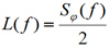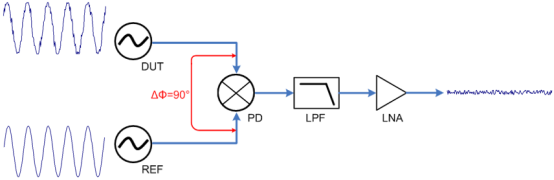News Category

# Two definitions of phase noise and brief description of test methods

Views : 37
Author : AmpliVsionS
Update time : 2021-06-23 16:22:22

Phase noise is a very important radio frequency index. In a communication system, phase noise will affect the vector modulation error of the vector modulation signal and worsen the bit error rate. In radar applications, phase noise will affect the radar's coherent processing gain and clutter suppression capabilities. In high-speed digital circuits, the jitter caused by phase noise also affects the maximum operating frequency of the digital circuit.

Phase noise is a measure of the frequency stability of an oscillator in a short period of time. The ideal sine wave signal can be expressed as follows:

A (t) =A0 cos(ω0 t +φ)

In real situations, amplitude, frequency, and phase may all fluctuate due to noise. Mathematically, frequency fluctuations and phase fluctuations can be combined into one item, which is uniformly represented by phase fluctuations. The real sine wave signal can be expressed as follows:

A (t) =A0 [1+α(t)] cos[ ω0 t +φ(t)]

Among them, α(t) is the amplitude fluctuation, and φ(t) is the phase fluctuation. Both amplitude noise and phase noise will cause the signal spectrum to broaden.

1. The spectrum definition and test method of phase noise

The description of phase noise is generally not described in the time domain, but in the frequency domain, which can describe the phase noise at different frequency offsets from the carrier.

The traditional phase noise is defined as follows: the relative noise power of the single sideband at a certain frequency offset with the amplitude of the carrier as a reference. This value refers to the relative noise level in a bandwidth of 1 Hz, and its unit is dBc/Hz. We can call it the spectral definition of phase noise.Figure 1. Schematic diagram of the spectrum definition of phase noise

This definition of phase noise can be easily tested with a spectrum analyzer, so it is also the most widely known definition of phase noise. In the 1988 version of the "IEEE standard definition of basic frequency and time measurement of physical quantities" (IEEE standard 1139-1988) also adopted this definition.

However, there are many limitations in measuring phase noise with a spectrum analyzer:

a. The measurement sensitivity is not high and is limited by the inherent phase noise of the spectrum analyzer. Because the spectrum analyzer is a superheterodyne receiver structure, the phase noise of the receiver's local oscillator limits the sensitivity of the spectrum analyzer to measure phase noise.

b. It is not possible to distinguish between AM noise and phase noise, because both will cause a broadening of the spectrum.

c. The phase noise of very close carriers cannot be measured, and the minimum frequency deviation is limited by the shape factor of the resolution filter.

d. The dynamic range of the spectrum analyzer also limits the phase noise measurement sensitivity. Due to the huge difference between noise power and carrier power, the dynamic range of the spectrum analyzer also limits the phase noise measurement sensitivity.

2. Phase definition and test method of phase noise

In order to measure phase noise with higher sensitivity, engineers improved the method of phase noise testing, and also changed the definition of phase noise. This new measurement method is the phase detector method that directly measures the phase of the signal.

In the 1999 edition of the "IEEE standard definition of basic frequency and time measurement physical quantities" (IEEE standard 1139-1999), the definition of phase noise was revised as: single-sideband phase noise L(f) is defined as random phase fluctuation φ( t) Half of the single-sideband power spectral density Sφ (f), and its unit is dBc/Hz. We can call it the phase definition of phase noise.This definition reverts to the physical quantity of phase fluctuation φ(t), using the frequency domain expression, and the coefficient of 1/2 is to be consistent with the result of the previous definition.

In the phase detector method, the phase time domain fluctuation of the signal is directly measured by the phase detector, and the phase noise described in the frequency domain is obtained through FFT transformation.Figure 2. Phase noise measurement with phase detector method

The phase detector method can be combined with a phase-locked loop to automatically lock the signal to be tested to realize automatic testing. In addition, the phase detector method can be combined with the cross-correlation algorithm, using two sets of independent hardware to greatly improve the measurement sensitivity of phase noise.

The advantages of the phase detector method to measure phase noise are:

· Can distinguish between AM noise and phase noise

· The sensitivity of measuring phase noise is greatly improved, and the cross-correlation algorithm can break through the limit of the meter's own phase noise. The carrier suppression effect of the phase detector can avoid the dynamic range problem.

· Can measure the phase noise of the close-in carrier.

3. The difference between the two definitions

When an RF engineer measures phase noise, it is likely that both definitions will be touched. When using a spectrum analyzer to test, it is based on the spectrum definition of phase noise; when using a more professional phase noise analyzer (or called a signal source analyzer) to test, it is based on the phase definition of phase noise.

In fact, when the phase noise is small, the measurement results obtained by the two definitions are consistent. In general engineering, the situation where phase noise needs to be measured is relatively small, so there is no need to worry about the measurement difference between the two definitions. According to engineering experience, when the phase noise is less than -80dBc/Hz, the measurement difference between the two definitions can be ignored.

However, the two definitions are not indistinguishable. The new phase definition has replaced the traditional spectrum definition, and the new definition is more concise in mathematics and returns to the essence of physical quantities. At the same time, the new phase definition does not exclude the case where the phase noise is greater than 0 dBc/Hz. Next time, if the measurement result of the close-in phase noise is greater than 0 dBc/Hz, don't be too surprised.